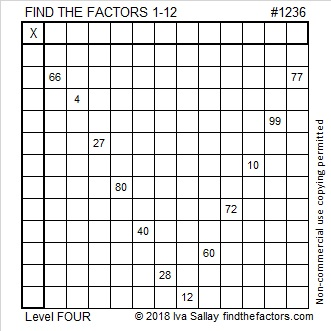# 1236 and Level 4

V is for victory. Can you be victorious solving this puzzle? Write the numbers from 1 to 12 in both the first column and the top row so that the puzzle functions like a multiplication table with the given clues becoming the products of the factors you write. I’m sure you can do it if you stick with it!Print the puzzles or type the solution in this excel file: 12 factors 1232-1241

Now I’ll tell you some facts about the number 1236:

• 1236 is a composite number.
• Prime factorization: 1236 = 2 × 2 × 3 × 103, which can be written 1236 = 2² × 3 × 103
• The exponents in the prime factorization are 2, 1, and 1. Adding one to each and multiplying we get (2 + 1)(1 + 1)(1 + 1) = 3 × 2 × 2 = 12. Therefore 1236 has exactly 12 factors.
• Factors of 1236: 1, 2, 3, 4, 6, 12, 103, 206, 309, 412, 618, 1236
• Factor pairs: 1236 = 1 × 1236, 2 × 618, 3 × 412, 4 × 309, 6 × 206, or 12 × 103
• Taking the factor pair with the largest square number factor, we get √1236 = (√4)(√309) = 2√309 ≈ 35.156791236 is the sum of consecutive prime numbers three rather interesting ways:

1. It is the sum of the twenty-two prime numbers from 13 to 103.
2. It is the sum of the eight prime numbers from 137 to 173.
(137 + 139 + 149 + 151 + 157 + 163 + 167 + 173 = 1236)
3. It is also the sum of twin primes: 617 + 619 = 1236

This site uses Akismet to reduce spam. Learn how your comment data is processed.